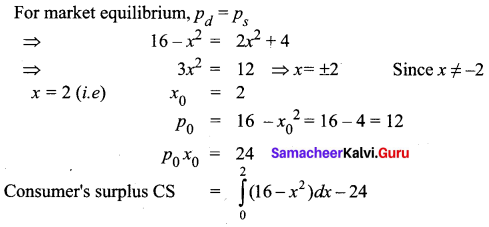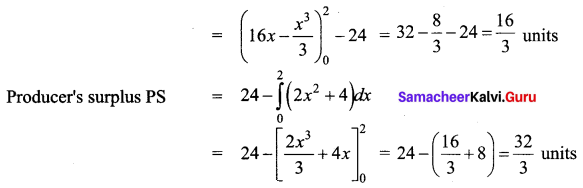# Samacheer Kalvi 12th Business Maths Solutions Chapter 3 Integral Calculus II Additional Problems

I. One Mark Questions

Question 1.
The area bounded by the curve y = ex, the x-axis and the lines x = 0 and x = 3 is _______
(a) e3 – 1
(b) e3 + 1
(c) e3
(d) e3 – 2
(a) e3 – 1
Hint: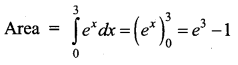Question 2.
The area bounded by the demand curve xy = 1, the x-axis, x = 1, x = 5 is ______
(a) log 5
(b) log $$\frac{1}{5}$$
(c) log 4
(d) $$\frac{1}{5}$$ log 2
(a) log 5
Hint: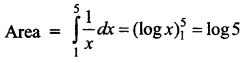Question 3.
If the marginal cost function MC = 7 – 4x then the cost function is _______
(a) 7x – 2x2 + k
(b) 7 – 4x2
(c) $$\frac{7}{x}$$ – 4
(d) 7x – 4x2
(a) 7x – 2x2 + k
Hint:
C = ∫MC dx
= ∫(7 – 4x) dx
= 7x – 2x2 + k

Question 4.
The marginal revenue R'(x) = $$\frac{1}{(x+1)}$$ Then the revenue function is _____
(a) $$\frac{-1}{x+1}$$
(b) $$\frac{1}{(x+1)^{2}}$$
(c) $$\log \frac{1}{(x+1)}$$
(d) log|x + 1| + k
(d) log|x + 1| + k
Hint:
Revenue function R = $$\int \frac{1}{x+1} d x$$ = log|x + 1| + k

Question 5.
Match the following.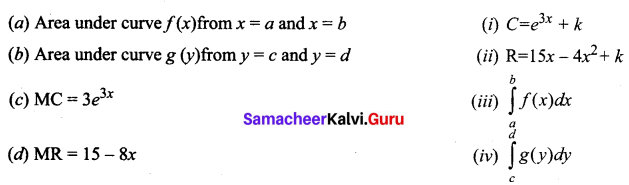(a) – (iii)
(b) – (iv)
(c) – (i)
(d) – (ii)

Question 6.
If demand function p = 35 – 2x – x2 and demand x0 = 3, then market price p0 is ________
p0 = 35 – 2(3) – 32
= 35 – 6 – 9
= 20

Question 7.
If supply law is p = 4 – x + x2 and p0 = 6, then demand x0 is ________
x2 – x + 4 = 6
⇒ x2 – x – 2 = 0
⇒ (x – 2) (x + 1) = 0
⇒ x = 2

Question 8.
Say True or False.
(a) Average cost function AC = $$\frac{\mathrm{MC}}{x}$$
(b) Total cost function C = ∫(MC) dx + k
(c) Demand function = P = Rx
(d) Elasticity of demand $$\eta_{d}=\frac{-p}{x} \frac{d x}{d p}$$
(e) Under market equilibrium x0 = p0
(a) False
(b) True
(c) False
(d) True
(e) False

II. 2 Mark Questions

Question 1.
Find the area bounded by the curve y2 = x3 and the lines x = 0, y = 1 and y = 2
Solution: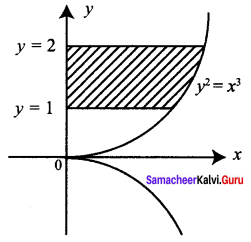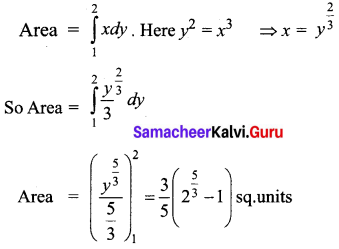Question 2.
Find the area bounded by one arch of the curve y = sin ax and the x-axis.
Solution: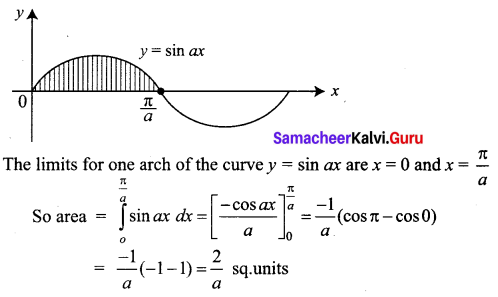Question 3.
The marginal cost of a commodity is 3x2 – 2x + 8. If there is no fixed cost find the total cost.
Solution:
C = ∫(MC) dx + k
= ∫(3x2 – 2x + 8) dx + k
= x3 – x2 + 8x + k
No fixed cost ⇒ k = 0
So total cost, C = x3 – x2 + 8x

Question 4.
For the marginal revenue function MR = 3 – 2x – x2, find the revenue function and demand function.
Solution: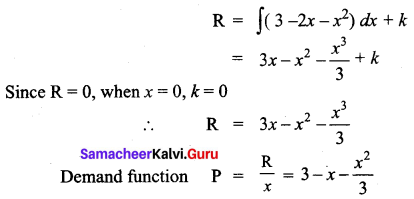Question 5.
The marginal cost at a production level of x units is C'(x) = 85 + $$\frac{375}{x^{2}}$$. Find the cost of producing 10 extra units, after 15 units have been produced.
Solution: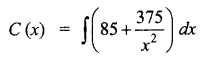But 15 units have been produced. We have to find the extra cost for 10 units that is, from 15 to 25 units. Hence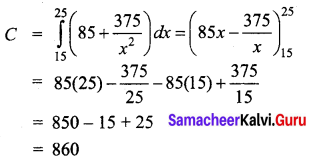Required cost is ₹860

III. 3 and 5 Marks Questions

Question 1.
Find the area under the curve y = 4x2 – 8x + 6 bounded by the y-axis, x-axis and the ordinate at x = 2.
Solution:
The shaded area is given by $$\int_{0}^{2} y d x$$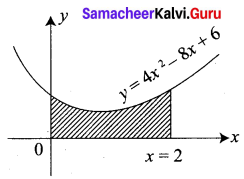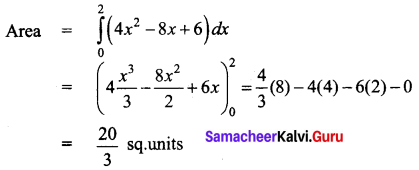Question 2.
The marginal revenue for a commodity is MR = $$\frac{e^{x}}{100}+x+x^{2}$$. Find the revenue function.
Solution: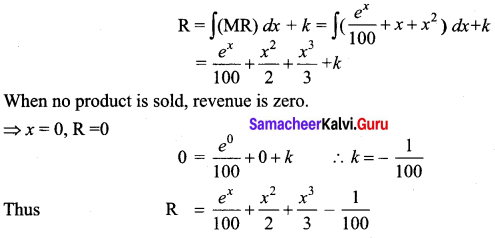Question 3.
The elasticity of demand with respect to price p is $$\frac{x-5}{x}$$ x > 5. Find the demand function if the price is 2 when demand is 7. Also find the revenue function.
Solution: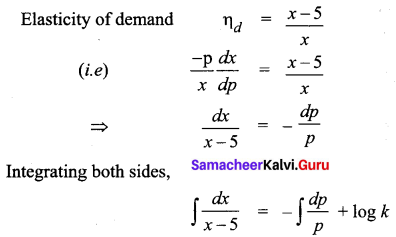⇒ log (x – 5) = -log p + log k
⇒ log (x – 5) + log p = log k
⇒ p (x – 5) = k
When p = 2, x = 1 ⇒ k = 4
Demand function is P = $$\frac{4}{x-5}$$
Revenue R = px or R = $$\frac{4x}{x-5}$$, x > 5

Question 4.
The marginal cost and marginal revenue of a commodity are given by C'(x) = 4 + 0.08x and R'(x) = 12. Find the total profit, given that the total cost at zero output is zero.
Solution:
Given that, MC = 4 + 0.08x
C(x) = ∫(4 + 0.08x) dx + k1
= 4x + 0.08$$\frac{x^{2}}{2}$$ + k1
= 4x + 0.04x2 + k1
But given when x = 0, C = 0, So k1 = 0
C(x) = 4x + 0.04x2
Now given that MR = 12
R(x) = ∫12 dx + k2 = 12x + k2
Revenue R = 0 when x = 0 ⇒ k2 = 0
R(x) = 12x
Total profit function P(x) = R(x) – C(x)
= 12x – 4x – 0.04x2
= 8x – 0.04x2

Question 5.
The marginal cost C'(x) and marginal revenue R'(x) are given by C'(x) = 20 + $$\frac{x}{20}$$ and R’ (x) = 30. The fixed cost is ₹ 200. Find the maximum profit.
Solution: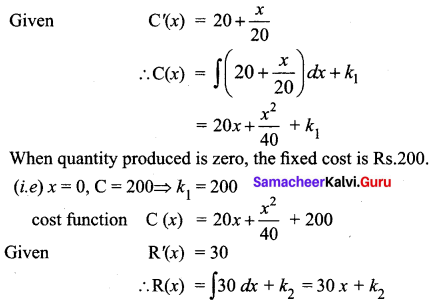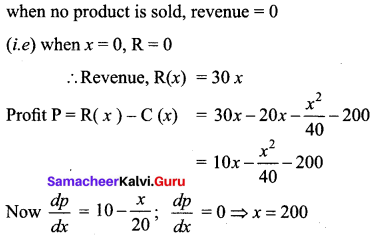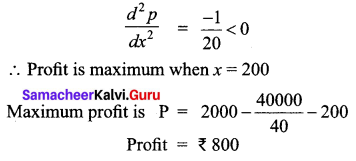Question 6.
The marginal cost of producing x units is C'(x) = 10.6x. The fixed cost is ₹ 50. The selling price per unit is Rs.5. Find (i) total cost function
(ii) total revenue function and
(iii) profit function.
Solution:
Given C'(x) = 10.6x
C'(x) = ∫(10.6x) dx + k
= 10.6$$\frac{x^{2}}{2}$$ + k
= 5.3x2 + k
The fixed cost is ₹ 50
(i.e.) when x = 0, c = 50 ⇒ k = 50
Hence cost function C = 5.3x2 + 50
Now, total revenue = number of units sold × price/unit
Since x be the number of units sold.
The selling price is Rs.5 per unit.
Revenue R(x) = 5x
Profit P = Total revenue – Total cost
= 5x – (5.3x2 + 50)
= 5x – 5.3x2 – 50

Question 7.
The demand and supply functions under pure competition are pd = 16 – x2 and ps = 2x2 + 4. Find the consumer’s surplus and producers’ surplus at the market equilibrium price.
Solution: